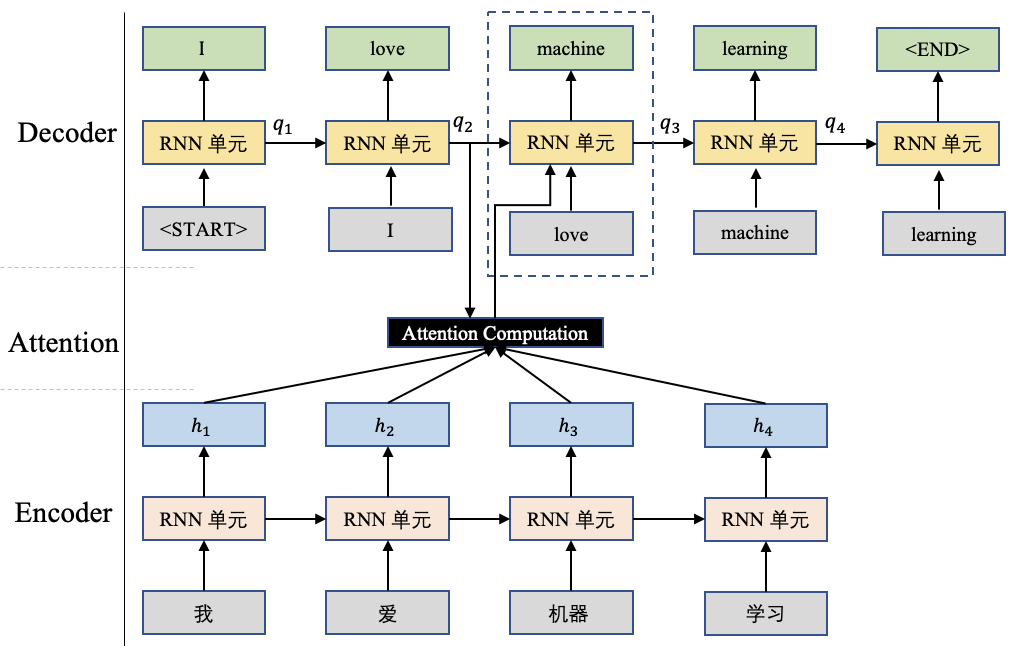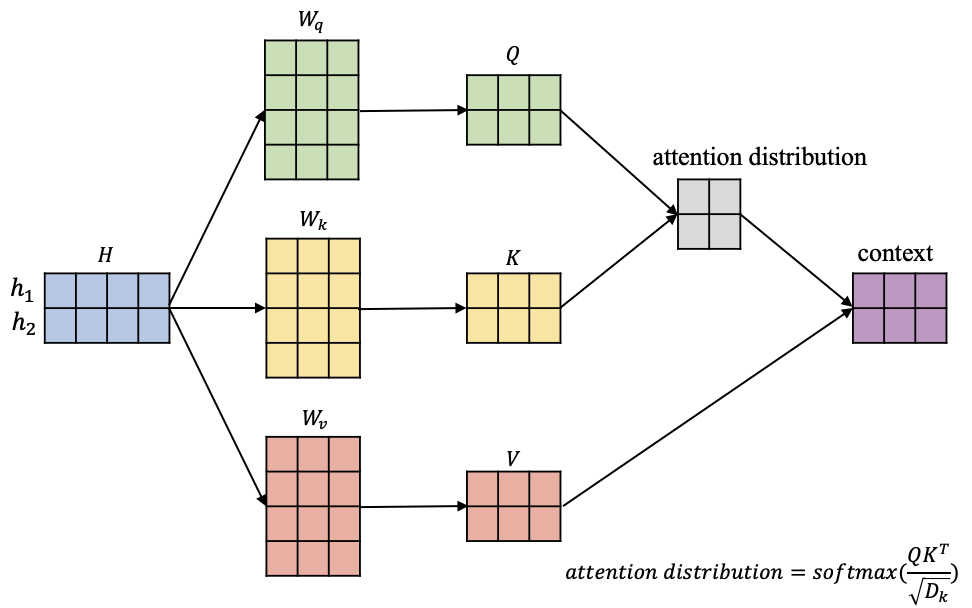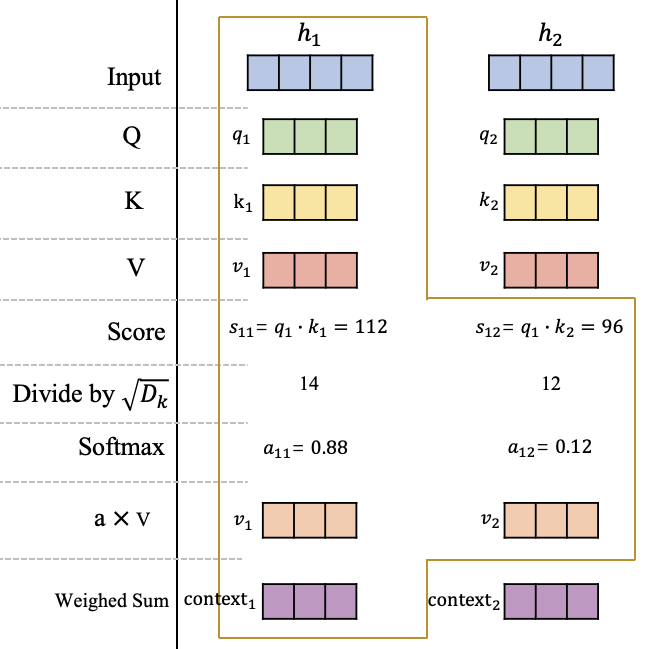# 自注意力机制¶$\begin{split} \left[ \begin{matrix} q_1 = h_1W_q \\ q_2=h_2W_q \end{matrix} \right] \Rightarrow Q=HW_q \end{split}$
$\begin{split} \left[ \begin{matrix} k_1 = h_1W_k \\ k_2=h_2W_k \end{matrix} \right] \Rightarrow K=HW_k \end{split}$
$\begin{split} \left[ \begin{matrix} v_1 = h_1W_v \\ v_2=h_2W_v \end{matrix} \right] \Rightarrow V=HW_v \end{split}$$\begin{split} Q=HW_q =[q_1,q_2,...,q_n] \\ K=HW_k =[k_1,k_2,...,k_n]\\ V=HW_v =[v_1,v_2,...,v_n] \end{split}$

$context_i=\sum_{j=1}^n softmax(s(q_i, k_j)) \cdot v_j$

$context=softmax(\frac{QK^T}{\sqrt{D_k}})V$